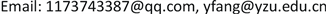OJCSOpen Journal of Circuits and Systems2327-0853Scientific Research Publishing10.12677/OJCS.2019.82004OJCS-30617OJCS20190200000_62808770.pdf信息通讯 工程技术 一种漏电流检测快速保护方法研究 Study on Leakage Current Detection and Fast Protection Method 亚华1212维轩1212正群12null扬州大学信息工程学院，江苏 扬州1904201908022233© Copyright 2014 by authors and Scientific Research Publishing Inc. 2014This work is licensed under the Creative Commons Attribution International License (CC BY). http://creativecommons.org/licenses/by/4.0/Copyright © 2019 by author(s) and Hans Publishers Inc.1. 引言

2. 漏电流检测和保护方法2.1. 漏电流检测电路工作原理

1) 自激振荡电路工作原理

2) 二阶有源低通滤波电路工作原理

A ( s ) = V o ( s ) V i ( s ) = A V F 1 + ( 3 − A V F ) s C R + ( s C R ) 2 (1)

A V F 1 = 1 + R 54 R 56 (2)

f c 1 = 1 2 π C 51 ⋅ C 53 ⋅ R 11 ⋅ R 51 (3)

3) 微分检测保护电路的工作原理

2.2. 漏电流检测保护原理

1) 漏电流较小时的响应

2) 漏电流较大时的响应

3. 漏电流检测保护电路的设计3.1. 自激振荡器的设计

1) 磁芯选取

2) 计算原副边绕组匝数

A e = ( OD-ID ) ⋅ HD = 20   mm 2 (4)

Q = π ⋅ ID 2 4 = 176.7   mm 2 (5)

N 1 = V c ⋅ T s 4 B m ⋅ A e = 73.171 (6)

3) 线径选取

I N 1 = I N 3 N 1 ⋅ N 3 = 4.11   mA (7)

n n 1 = I N 1 J ⋅ S d 3 = 0.058   m − 2 ⋅ kg − 1 ⋅ s 2 (8)

n n 2 = 50 ⋅ 10 − 3 J ⋅ S d 3 = 0.707   m − 2 ⋅ kg − 1 ⋅ s 2 (9)

n n 3 = 25 J × S d 4 = 6.25   m − 2 ⋅ kg − 1 ⋅ s 2 (10)

k = N 1 ⋅ n n 1 ⋅ S d 3 + N 2 ⋅ n n 2 ⋅ S d 3 + 4 N 3 ⋅ n n 3 ⋅ S d 4 Q = 0.078 (11)

3.2. 二阶有源低通滤波器的设计

A V F 1 = 1 + R 54 R56 = 2 (12)

f c 1 = 1 2 π C51 ⋅ C53 ⋅ R11 ⋅ R51 = 1.071   kHz (13)

A V F 2 = 1 + R55 R57 = 2.538 (14)

f c 2 = 1 2 π C52 ⋅ C54 ⋅ R 52 ⋅ R53 = 1.329   kHz (15)

A V F = A V F 1 ⋅ A V F 2 = 5.077 (16)

3.3. 漏电流检测微分保护电路参数设计

{ u c ( 80   μs ) = 12 ⋅ ( 1 − e − 80 ⋅ 10 − 6 τ 1 ) ≥ 0.6   V u c ( 80   μs ) ≥ 3.3   V (17)

τ 1 = R 3 ⋅ C 2 (18)

τ 1 = R 3 ⋅ C 2 ≤ 2.5 ⋅ 10 − 4 (19)

u c ( 160   μs ) = u c ( 80   μs ) e − 80 ⋅ 10 − 6 τ 2 ≥ 3.3   V (20)

τ 2 = ( R 3 + R 2 ) ⋅ C 2 (21)

τ 2 = ( R 3 + R 2 ) ⋅ C 2 ≥ 1.16 ⋅ 10 − 4 (23)

4. 实验结果

5. 结论